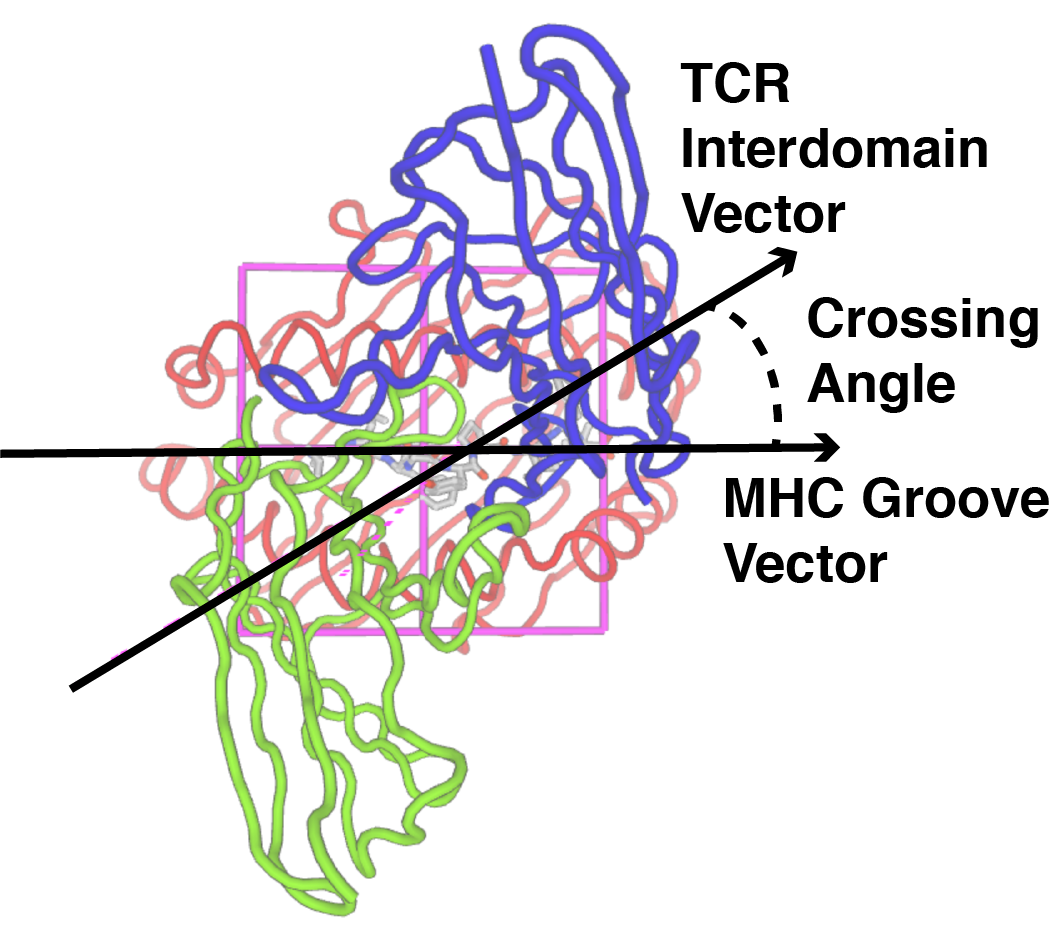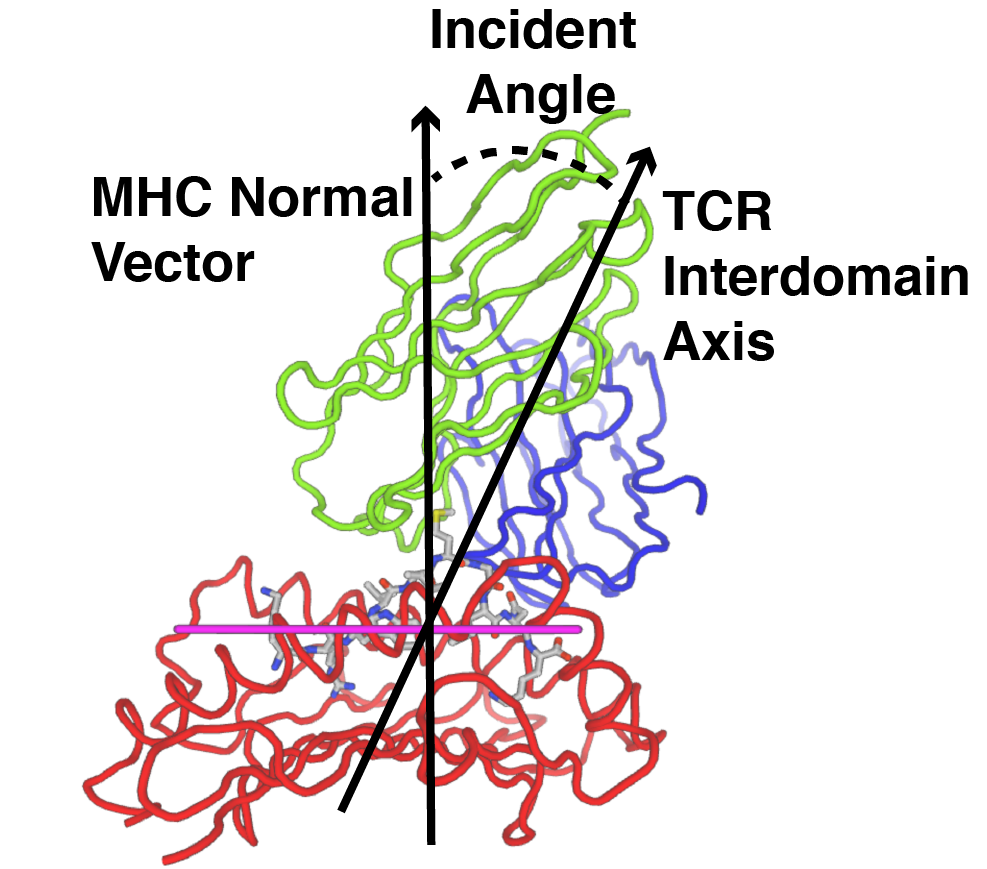TCR3d
T cell receptor structural repertoire database

## Crossing and Incident Angles

### Crossing or Docking Angle (TCR Twist)

The crossing or docking angle is the angle between the MHC pocket (peptide binding groove) and the vector between the TCR domains; the latter is calculated using the centroids of the conserved disulfide bonds in each domain. This corresponds to the twist of the TCR over the peptide-MHC, and its calculation here follows the methodology described by Rudolph, Stanfield, Wilson (Annu. Rev. Immunol. 2006).Shown above is the A6 TCR binding to Tax peptide and HLA-A2 MHC (1AO7), with crossing angle of 33.5 degrees. Colors are: TCR alpha, TCR beta, MHC, peptide, MHC plane.

### Incident Angle (TCR Tilt)

The incident or tilt angle is the angle between the MHC peptide groove plane normal vector and the TCR interdomain axis of rotation. This corresponds to the degree of tilt of the TCR over the peptide-MHC.Shown above is the C12C TCR binding to HIV peptide and HLA-B27 MHC (4G9F), with incident angle of 20.6 degrees. Colors are: TCR alpha, TCR beta, MHC, peptide, MHC plane.